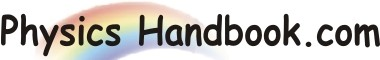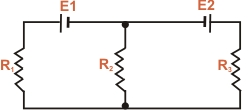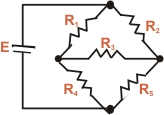HOME TOPICS DEFINITIONS TABLES LAWS INVENTIONS EXPERIMENTS QUIZ VIDEOS
 A B C D E F G H I J K L M N O P Q R S T U V W X Y Z
Complex Circuits
Sometimes we encounter circuits where simplification by series and parallel combinations is impossible. Consequently, Ohm's law cannot be applied to solve such circuits. This happens when there is more than one source of e.m.f. in the circuit or when resistors are connected in a complicated manner. such circuit are called complex circuits. We shall discuss two such circuits by way of illustration.
(I) Fig (a) shows a circuit containing two source of e.m.f. E1 and E 2 are three resistors. This circuit cannot be solved by series-parallel combinations. Are resistor R 1 and R 3 in series? Not quite, because same current does not flow in them.Fig (a)
(II) Fig (b) shows another circuit where we cannot solve the circuit by using series and parallel combinations. Through this contains one source of e.m.f. (E), it cannot be solved by using series and parallel combinations. Are resistors R 1 and R 2 are neither in series nor in parallel, same is true for other pair of resistors.Fig (b)
In order to solve such complex circuits, Gustav Kirchhoff gave two laws , known as Kirchhoff's laws.